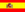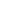home | más chusmeríos | otros temas de Física | lecciones del maestro Ciruela | tonterías | @NO ME SALEN
THEORETICAL NOTES AND EXCERCISES OF PHYSICS AND BIOPHYSICSMiscellany
Passage units
MULTIPLICATION BY A CONVERSION FACTOR

This is the method I recommend NOT using; Nonetheless, I acknowledge of it´s popularity, specially among the engineers, who love to make classification charts of every piece of crap they find in the universe.

It consists in finding a factor that converts a unit (or a group of units) in other (or others). The factors for simple conversions appear in textbooks; With them, compound factors are made, these ones swarm in the cheat sheets of the CBC students. Leave them be.

 Example 1) convert 20 m/s to km/h To convert from m/s to km/h you only need to multiply by 3.6 this comes from dividing 0,001 (the factor used to convert m to km) by 0,0002778 ((the factor used to convert s to h), this way: 20 m/s = 20 . 3.6 km/h 20 m/s = 72 km/h Example 2) convert 3,500 km/h² to m/s² To convert from m/s to km/h you only need to multiply by 0.00007716 this comes from dividing 1,000 (the factor used to convert km to m) by 12,960,000 (the factor used to convert h² to s²), this way: 3,500 km/h² = 3,500 . 0.00007716 . m/s² 3,500 km/h² = 0.27 m/s² Example 3) convert 0,7 kgf/cm to N/m To convert from kgf/cm to N/m only need to multiply by 1,000 this comes from dividing 10 (the factor used to convert  kgf a N) by 0,01 (the factor used to convert cm to m), this way: 0.7 kgf/cm = 0.7 . 1,000 . N/m 0.7 kgf/cm = 700 N/m Example 4) convert 60.000  J/day to W To convert from J/day to W you only need to multiply by 0.000011574 -or wat is the same: divide by 86,400-, wich comes from the amount of seconds there are on a sunny day. 60,000  J/day = 60,000 . 0.000011574 . W 60,000  J/day = 0.694 W The inverse multiplicative of any conversion factor is the inverse conversion´s factor (what a tongue-wister!). That´s how 3.6 is the factor to convert m/s to km/h, then, dividing by 3.6 or multiplying by 0.2778 will be the right thing to do if you want to convert km/h to m/s. I sware it is like this. You will have a beautifull cheat sheet.Some rights reserved. Reproduction allowe by quoting the source. Translated by Santi Pisci. Last Updated jul-15. Buenos Aires, Argentina.